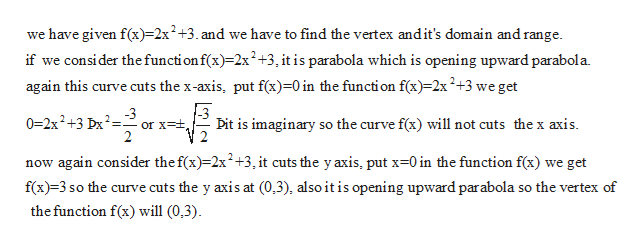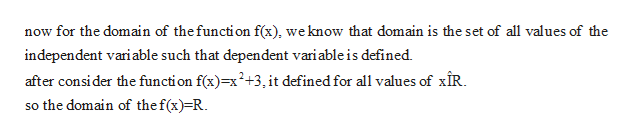# f(x)=2x2+3What is the vertex?What is the domain and range?

Question
3 views

f(x)=2x2+3

What is the vertex?

What is the domain and range?

check_circle

Step 1

vertex of the opening upward parabola.help_outlineImage Transcriptionclosewe have given f(x)-2x+3.and we have to find the vertex andit's domain and range. if we consider the function f(x)-2x2+3, it is parabola which is opening upward parabola again this curve cuts the x-axis, put f(x)=0 in the function f(x)-2x2+3 we get -3 or xt 2 0=2x2+3 Dx2 Dit is imaginary so the curve f(x) will not cuts the x axis now again consider the f(x)2x2+3, it cuts the y axis, put x-0 in the function f(x) we get f(x) 3 so the curve cuts the y axis at (0,3), also it is opening upward parabola so the vertex of the function f(x) will (0,3) fullscreen
Step 2

domain of the f...help_outlineImage Transcriptionclosenow for the domain of thefunction f(x), we know that domain is the set of all values of the independent variable such that dependent variable is defined after consi der the functi on fx)=x2+3,it defined for all values of xîR so the domain of the f(x)=R fullscreen

### Want to see the full answer?

See Solution

#### Want to see this answer and more?

Solutions are written by subject experts who are available 24/7. Questions are typically answered within 1 hour.*

See Solution
*Response times may vary by subject and question.
Tagged in

### Algebra